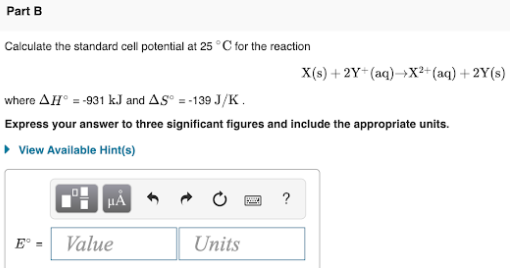# Calculate the standard cell potential at 25 °C for the reaction X(s) + 2Y^+(aq) → X^2(aq) + 2Y(s) where ΔH° = -931 kJ and ΔS° = -139 J/K. Express your answer to three significant figures and include the appropriate units.